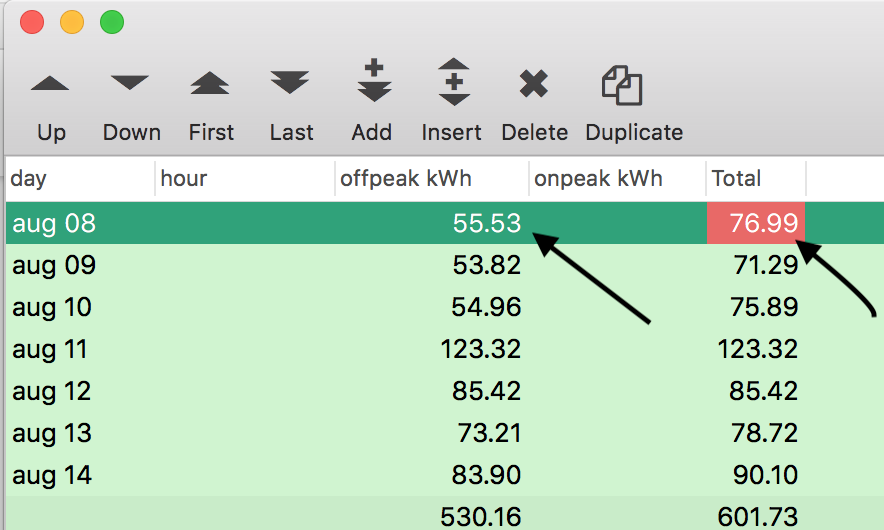# Calculate Results

I would like to create Percent based on two summary total

Perhaps you could go into a bit more detail?

I have summary of On_Peak data by Day and hour, Off_Peak data by Day and Hour, Totalized by Hour.
Now I would like to get a percentage of On_Peak by Day.

Sorry, I still don’t understand. Percentage of what? Percentage is just division. If you have values A and B, and you want to know the percentage of A relative to B, the formula is:

``````A*100/B
``````

Hmm, maybe what you want is

``On_Peak*100/Totalized``

The daily totals are summarized by day in Summarize and Analyze.
Can I use the summary totals to calculate the percentage?
I know what needs to be done, but I don’t know how it’s done in Panorama X.

Please, tell us. Calculate the percentage of what?

Do you want to calculate each day as a percentage of the total? If so, this short program will convert totals into percentages. This assumes that the field containing the values you want to turn into percentages has been clicked on. This code takes advantage of the fact that in a formula, «» can be used for the current field.

``````outlinelevel 1
lastrecord
let gtotal = «»
formulafill «»*100/gtotal``````

How much the daily energy is On_Peak?
The daily total is derived from the summary of hourly data by Day (this total is not in a field but calculated - summarized).

At that point, I will have (OnPeak/Total)*100 = Daily Percentage

Perhaps you could post a screenshot with arrows pointing to the OnPeak and Total hours?

Hi Jim,

Thanks for all of your help on this. It’s been a while, since I’ve used Panorama.

Electric Bill

The electric company collect hourly data for each day. The energy is either OnPeak or OffPeak. I created a Total field at the hourly level. I summarize the information using summarize and analyze.I want to calculate the daily off-peak percentage.
Using Aug 8 data Daily_Percentage = 55.53 / 76.99 * 100
Can I keep the results in any way?

Chuck

Why not create an Average field and keep it there?

James you mean create an actual field in the database and save it there.
This will be done at summary by day?

The hourly records will either total be 100% or 0%, it’s either on_peak or off_peak.

I’m a different James, but yes, that is what he means, and I was going to suggest that myself. Perhaps call the field `offpeak %`. After the summaries are calculated then you would use the formulafill statement to calculate the percentages.

``````field "Whatever your percentage field name is"
formulafill «offpeak kWh»*100/Total
``````

What you might want to do is use the procedure recorder to record the actions of the Summarize and Analyze dialog, then add the code above to the bottom of the recorded code. Then you would simply re-run the procedure to recreate the analysis whenever you need it, you won’t need to use the Summarize and Analyze dialog.

Your finished program is probably going to look something like this:

``````field day
groupup by day
field "offpeak kWh"
total
field Total
total
outlinelevel 1
field "offpeak %"
formulafill «offpeak kWh»*100/Total``````